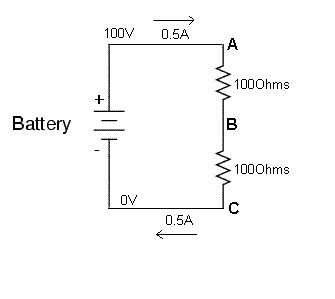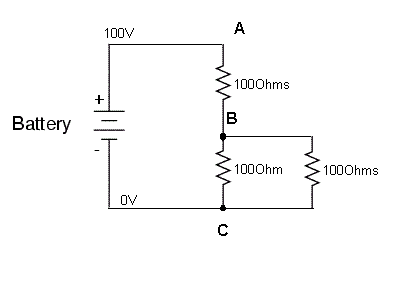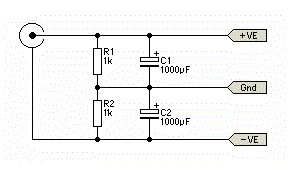# Questions about voltage divider circuits

• Planobilly
LEDs.I don't have a simple drawing program to draw the above circuit. Recommendations for a simple drawing program would help.Draw a circuit using LTspice and copy the bitmap to the clipboard.f

#### Planobilly

Say I had a 10VDC supply and I need around 3.3VDC at 30ma. Let's say the 3.3VDC will power a LED.

V1= 10VDC
R1= 82 ohms
R2= 47 ohms
V2= 3.64 VDC from the junction to ground
I= 28.24ma...I guess
I don't know what effect the LED will have on the circuit and I don't understand how to find the resistance of a LED.

I ASSUME in general the value or R1 and R2 in a voltage divider is based on the needed current through the load. I have zero idea of the effect of other components that could be in a circuit passed the LED supplied by the same 10VDC supply.

I don't have a simple drawing program to draw the above circuit. Recommendations for a simple drawing program would help.

Bottom line, as a concept: I ASSUME a voltage divider can be used to control both voltage and current. Is this correct.

Cheers,

Billy

Last edited:
Say I had a 10VDC supply and I need around 3.3VDC at 30ma. Let's say the 3.3VDC will power a LED.

just a single resistor is needed to power a LED
There are several styles of LED's each style has a specific voltage drop (Vd) across it and a required current

have a look at this electronics site that I am involved with. I has a good resource on driving LEDs

Dave

HI Dave,

I understand pretty well the LED voltages as they relate to different colors and understand about needing only one resistor to light a LED. I could have used a lamp in the same circuit.

My questions are more about voltage dividers and how and why they work.

Is my basic assumption that voltage dividers can control both voltage and current correct?

Billy

EDIT: The current in the above circuit is not correct correct

Last edited:
My questions are more about voltage dividers and how and why they work.

Is my basic assumption that voltage dividers can control both voltage and current correct?

OK sorry, your LED Q's comments threw me off the pathYes they control voltage and current, but in a very poor way, because any variation in the load resistance
will alter both the current and voltage

take a basic voltage divider cctsimple maths tell us that, because the resistor values are equal, the voltage at point B will be 50V ( 1/2 the supply voltage)

now add another 100 Ohm resistor as a load and you can see that the total resistance between B and C has now changed
and this is going to completely alter the respective voltage drops across the to resistor and the paralleled bottom pair
and also change the current. And any minor changes of that 100 Ohm ( right one) is going to continuously change the whole set upDave

Hi Dave,

Yes, I understand everything you said. I have built two circuits. One with 83 ohm/47 ohm with 1/4 watt resistors...which got a bit hot...lol and another at 2 K/1 K with 1 watt resistors. On the 1 K/ 2 K divider I calculate I=3.333mA and the actual was 3.677mA. The difference I assume is the resistance of the diode and any out of value that may exist in the resistors.

I don't have a simple drawing program to draw the above circuit. Recommendations for a simple drawing program would help.
It will do your drawing and also simulate the circuit.
There is a Tool, "copy bitmap to clipboard" that will export diagrams.

I guess I better go to bed. I have to rebuild a 1941 seven stage super heterodyne radio tomorrow.

Thanks to both of you for the instructions. I have fair skills at repairing tube guitar amps and radios but I am still learning the basics of electronics.

Cheers,

Billy

•davenn
An LED is not a resistive device and you can't just assume it will behave like the 'bottom' resistor in a resistive divider. Read what Baluncore has written, above. It is probably best to look at the spec of your LED and see what Voltage it needs to operate on. Then calculate the resistance needed to drop the supply volts to that which the LED needs, when the specified current flows through the LED. It's easy enough to do and avoids the problem that the simple Potential Divider sums will not help you directly.
V across the dropping resistor = V supply - V LED
then
R = V across the dropping resistor/ ISpecified

•jim hardy
Sorry guys...this thread got to be about LEDs and my only real questions were about voltage dividers in concept.

Thanks,

Billy

•davenn
Sorry guys...this thread got to be about LEDs and my only real questions were about voltage dividers in concept.

Thanks,

Billy

no probs
how did the radio rebuild go ?

one thing I forgot to mention the other day about voltage dividers is and is REALLY handy if you need a basic split rail supply
from a battery or other single rail source ...

another of my quick drawings ...Dave

#### Attachments

Thanks Dave,

Thanks for the drawings.

The radio rebuild went without any issues. There are good schematics of old radios. I never have much problem with old radios. There are a few parts from time to time that prove hard to find. Every now and then I wind up cranking up the CNC machine to make something but not often.

Current project is fixing a guitar amp kit for a kid to prevent him from killing himself. I never say too much about safety but after seeing the mess this kid made I had to give him a good talking to. Not sure it did any good but some things have to be said. The transformer puts out 731 VAC top to bottom and 367 VAC to the center tap. Serious voltage!

Cheers,

Billy

•davenn